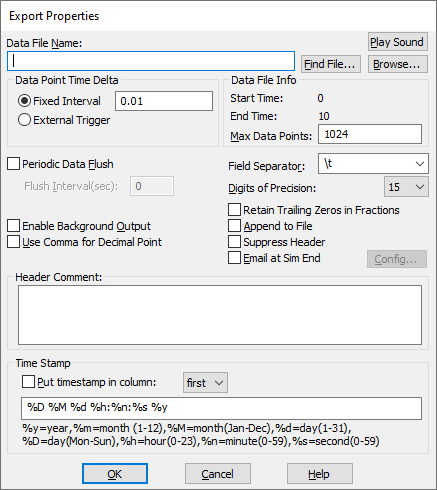## less than or equal to (<=)Block Category: Boolean

Inputs: Real, complex, or fixed-point scalars, or vectors or matrices.

l: numerator x1.

r: denominator x2

Description: The <= block generates an output signal of 1 if and only if input signal x1 is less than or equal to input signal x2. Otherwise, the output is 0.

The <= block accepts matrix operands and generates a result matrix of 0s and 1s.

Right-click the <= block to assign a different function to the block.

#### Example

1. Simple if-then-else construct

Consider a variable y such that:

If x ≤ 0.5 then y = cos(3t); else y = 0

where t is simulation time. Let x be a unit step delayed by 7 sec, represented as u(- 7). This system can be realized as:Until the onset of the step input at t = 7 sec, the Boolean inequality x 0.5 evaluates to true, and y takes on a value of cos(3t). At  t = 7 sec, the Boolean inequality evaluates to false and remains false for the duration of the simulation. Consequently, from this point onwards, y takes on the value of 0. The lower plot block monitors the outputs of the cos and variable x blocks.